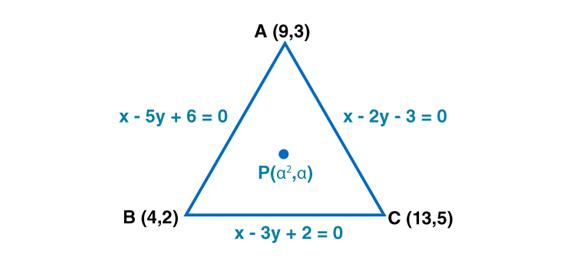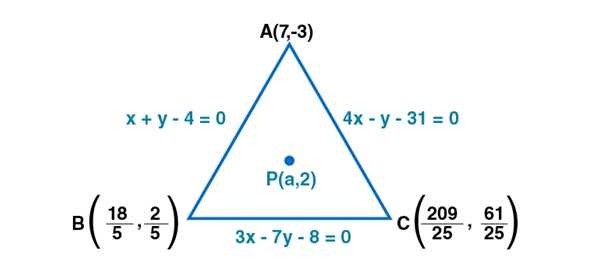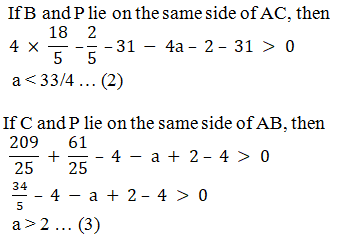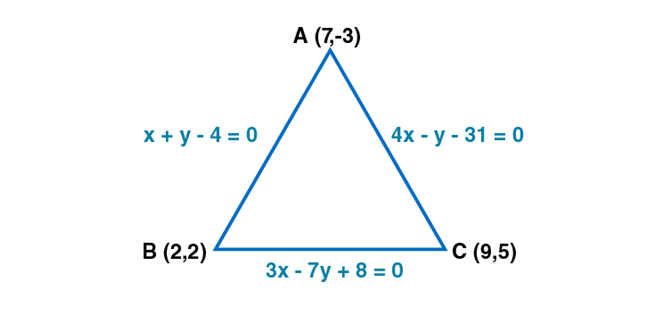• +91 9971497814
• info@interviewmaterial.com

# RD Chapter 23- The Straight Lines Ex-23.14 Interview Questions Answers

### Related Subjects

Question 1 : Find the values of α so that the point P(α 2, α) lies inside or on the triangle formed by the lines x – 5y + 6 = 0, x – 3y + 2 = 0 and x – 2y – 3 = 0.

Given:

x – 5y + 6 = 0, x – 3y+ 2 = 0 and x – 2y – 3 = 0 forming a triangle and point P(α2, α)lies inside or on the triangle

Let ABC be thetriangle of sides AB, BC and CA whose equations are x − 5y + 6 = 0,x − 3y + 2 = 0 and x − 2y − 3 = 0, respectively.

On solving theequations, we get A (9, 3), B (4, 2) and C (13, 5) as the coordinates of thevertices.It is given that pointP (α2, α) lies either inside or on the triangle. The threeconditions are given below.

(i) A and P must lieon the same side of BC.

(ii) B and P must lieon the same side of AC.

(iii) C and P must lieon the same side of AB.

If A and P lie on thesame side of BC, then

(9 – 9 + 2)(α2 –3α + 2) ≥0

(α – 2)(α –1) ≥ 0

α  (- ∞, 1 ]  [ 2, ∞) … (1)

If B and P lie on thesame side of AC, then

(4 – 4 – 3) (α2 –2α – 3) ≥ 0

(α – 3)(α +1) ≤ 0

α  [- 1, 3] … (2)

If C and P lie on thesame side of AB, then

(13 – 25 + 6)(α2 –5α + 6) ≥0

(α – 3)(α –2) ≤ 0

α  [ 2, 3] … (3)

From equations (1),(2) and (3), we get

α [2, 3]

α [2, 3]

Question 2 : Find the values of the parameter a so that the point (a, 2) is an interior point of the triangle formed by the lines x + y – 4 = 0, 3x – 7y – 8 = 0 and 4x – y – 31 = 0.

Given:

x + y – 4 = 0, 3x – 7y– 8 = 0 and 4x – y – 31 = 0 forming a triangle and point (a, 2)is an interiorpoint of the triangle

Let ABC be thetriangle of sides AB, BC and CA whose equations are x + y − 4 = 0,3x − 7y − 8 = 0 and 4x − y − 31 = 0,respectively.

On solving them, weget A (7, – 3), B (18/5, 2/5) and C (209/25, 61/25) as thecoordinates of the vertices.

Let P (a, 2) be thegiven point.It is given that pointP (a, 2) lies inside the triangle. So, we have the following:

(i) A and P must lieon the same side of BC.

(ii) B and P must lieon the same side of AC.

(iii) C and P must lieon the same side of AB.

Thus, if A and P lieon the same side of BC, then

21 + 21 – 8 – 3a – 14– 8 > 0

a > 22/3 … (1)From (1), (2) and (3),we get:

(22/3, 33/4)

(22/3, 33/4)

Question 3 : Determine whether the point (-3, 2) lies inside or outside the triangle whose sides are given by the equations x + y – 4 = 0, 3x – 7y + 8 = 0, 4x – y – 31 = 0.

Given:

x + y – 4 = 0, 3x – 7y+ 8 = 0, 4x – y – 31 = 0 forming a triangle and point (-3, 2)

Let ABC be thetriangle of sides AB, BC and CA, whose equations x + y − 4 = 0,3x − 7y + 8 = 0 and 4x − y − 31 = 0,respectively.

On solving them, weget A (7, – 3), B (2, 2) and C (9, 5) as the coordinates of the vertices.

Let P (− 3, 2) bethe given point.The given point P(− 3, 2) will lie inside the triangle ABC, if

(i) A and P lies onthe same side of BC

(ii) B and P lies onthe same side of AC

(iii) C and P lies onthe same side of AB

Thus, if A and P lieon the same side of BC, then

21 + 21 + 8 – 9 – 14 +8 > 0

50 × – 15 > 0

-750 > 0,

This is false

The point (−3, 2)lies outside triangle ABC.

Todays Deals### RD Chapter 23- The Straight Lines Ex-23.14 Contributorskrishan

Name:
Email:

# Latest News# 9000 interview questions in different categories